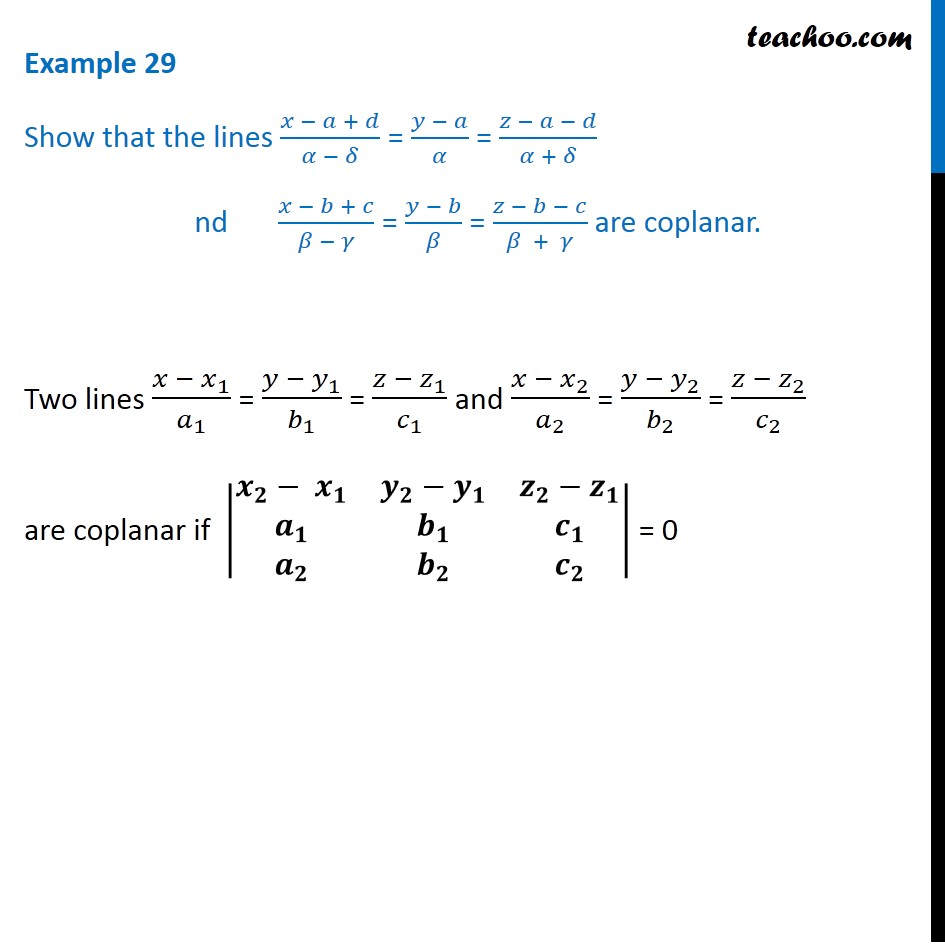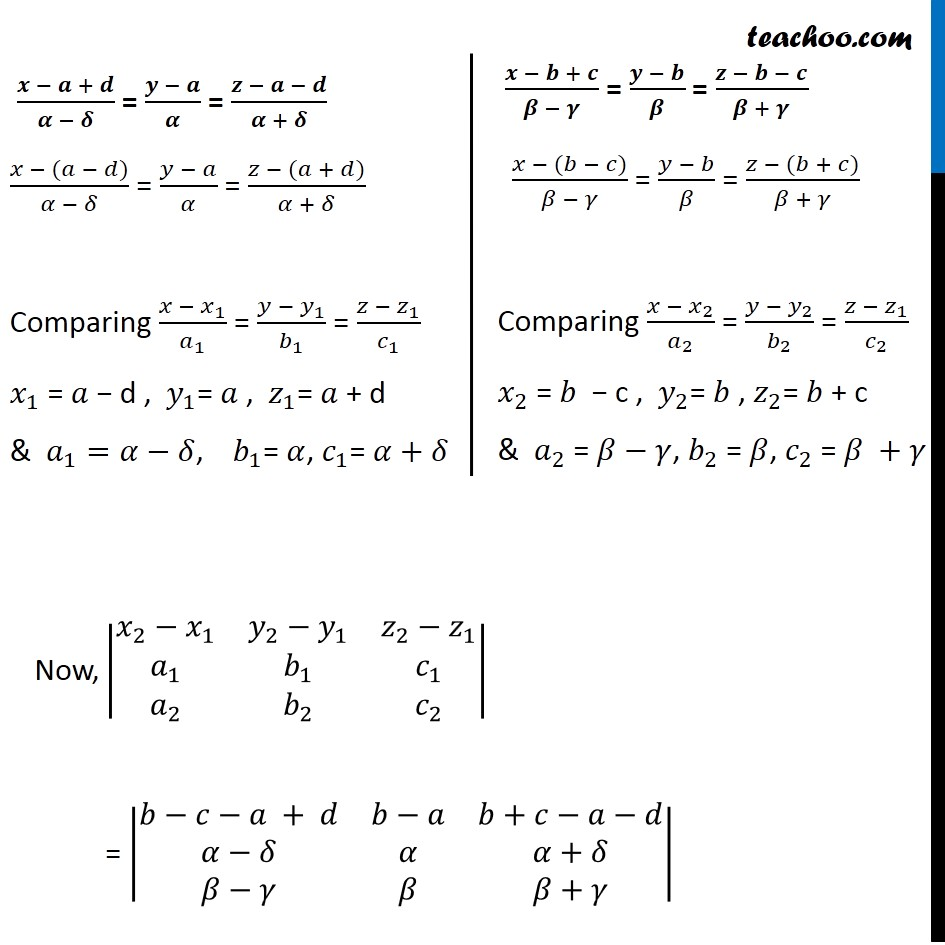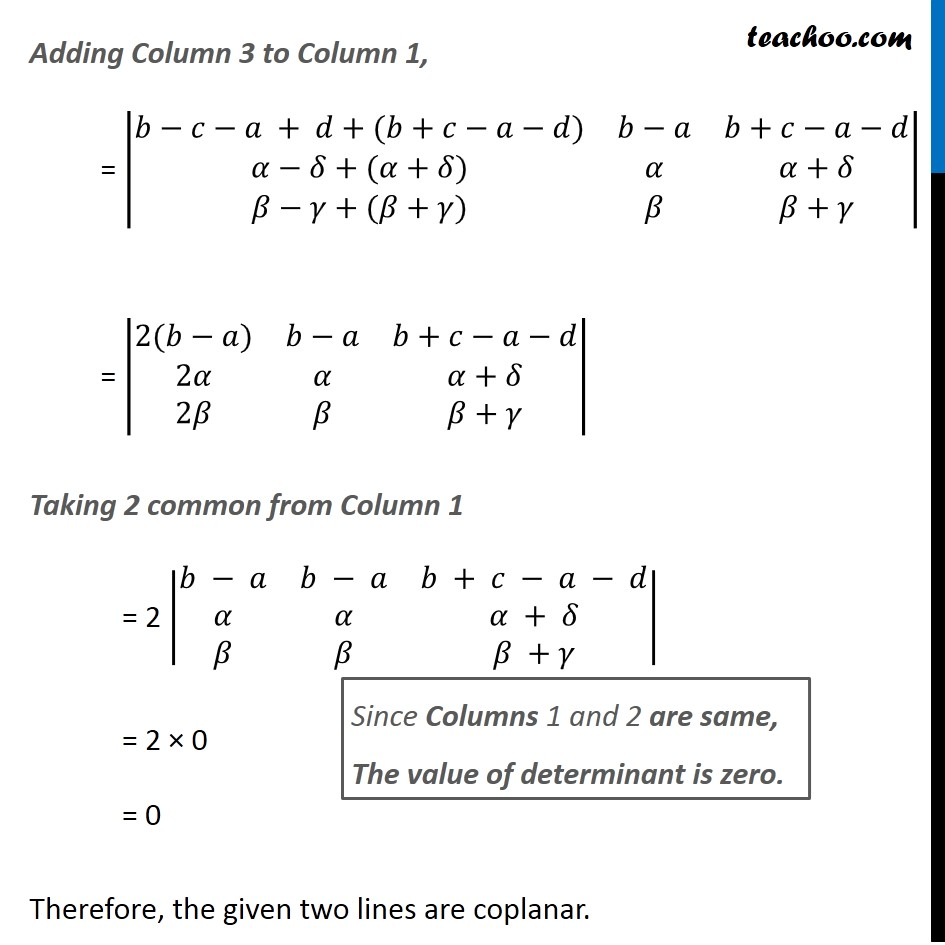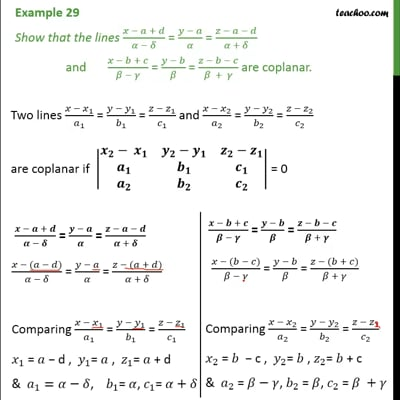Examples

Chapter 11 Class 12 Three Dimensional Geometry
Serial order wiseThis video is only available for Teachoo black users

Learn in your speed, with individual attention - Teachoo Maths 1-on-1 Class

### Transcript

Question 19 Show that the lines (𝑥 − 𝑎 + 𝑑)/(𝛼 − 𝛿) = (𝑦 − 𝑎)/𝛼 = (𝑧 − 𝑎 − 𝑑)/(𝛼 + 𝛿) nd (𝑥 − 𝑏 + 𝑐)/(𝛽 − 𝛾) = (𝑦 − 𝑏)/𝛽 = (𝑧 − 𝑏 − 𝑐)/(𝛽 + 𝛾) are coplanar.Two lines (𝑥 − 𝑥_1)/𝑎_1 = (𝑦 − 𝑦_1)/𝑏_1 = (𝑧 − 𝑧_1)/𝑐_1 and (𝑥 − 𝑥_2)/𝑎_2 = (𝑦 − 𝑦_2)/𝑏_2 = (𝑧 − 𝑧_2)/𝑐_2 are coplanar if |■8(𝒙_𝟐− 𝒙_𝟏&𝒚_𝟐−𝒚_𝟏&𝒛_𝟐−𝒛_𝟏@𝒂_𝟏&𝒃_𝟏&𝒄_𝟏@𝒂_𝟐&𝒃_𝟐&𝒄_𝟐 )| = 0 (𝒙 − 𝒂 + 𝒅)/(𝜶 − 𝜹) = (𝒚 − 𝒂)/𝜶 = (𝒛 − 𝒂 − 𝒅)/(𝜶 + 𝜹) (𝑥 − (𝑎 − 𝑑))/(𝛼 − 𝛿) = (𝑦 − 𝑎)/𝛼 = (𝑧 − (𝑎 + 𝑑))/(𝛼 + 𝛿) Comparing (𝑥 − 𝑥_1)/𝑎_1 = (𝑦 − 𝑦_1)/𝑏_1 = (𝑧 − 𝑧_1)/𝑐_1 𝑥_1 = 𝑎 − d , 𝑦_1= 𝑎 , 𝑧_1= 𝑎 + d & 𝑎_1=𝛼−𝛿, 𝑏_1= 𝛼, 𝑐_1= 𝛼+𝛿 (𝒙 − 𝒃 + 𝒄)/(𝜷 − 𝜸) = (𝒚 − 𝒃)/𝜷 = (𝒛 − 𝒃 − 𝒄)/(𝜷 + 𝜸) (𝑥 − (𝑏 − 𝑐))/(𝛽 − 𝛾) = (𝑦 − 𝑏)/𝛽 = (𝑧 − (𝑏 + 𝑐))/(𝛽 + 𝛾) Comparing (𝑥 − 𝑥_2)/𝑎_2 = (𝑦 − 𝑦_2)/𝑏_2 = (𝑧 − 𝑧_1)/𝑐_2 𝑥_2 = 𝑏 − c , 𝑦_2= 𝑏 , 𝑧_2= 𝑏 + c & 𝑎_2 = 𝛽−𝛾, 𝑏_2 = 𝛽, 𝑐_2 = 𝛽 + 𝛾 Now, |■8(𝑥_2−𝑥_1&𝑦_2−𝑦_1&𝑧_2−𝑧_1@𝑎_1&𝑏_1&𝑐_1@𝑎_2&𝑏_2&𝑐_2 )| = |■8(𝑏−𝑐−𝑎 + 𝑑&𝑏−𝑎&𝑏+𝑐−𝑎−𝑑@𝛼−𝛿&𝛼&𝛼+𝛿@𝛽−𝛾&𝛽&𝛽+𝛾)| Adding Column 3 to Column 1, = |■8(𝑏−𝑐−𝑎 + 𝑑+(𝑏+𝑐−𝑎−𝑑)&𝑏−𝑎&𝑏+𝑐−𝑎−𝑑@𝛼−𝛿+(𝛼+𝛿)&𝛼&𝛼+𝛿@𝛽−𝛾+(𝛽+𝛾)&𝛽&𝛽+𝛾)| = |■8(2(𝑏−𝑎)&𝑏−𝑎&𝑏+𝑐−𝑎−𝑑@2𝛼&𝛼&𝛼+𝛿@2𝛽&𝛽&𝛽+𝛾)| Taking 2 common from Column 1 = 2 |■8(𝑏 − 𝑎&𝑏 − 𝑎&𝑏 + 𝑐 − 𝑎 − 𝑑@𝛼&𝛼&𝛼 + 𝛿@𝛽&𝛽&𝛽 +𝛾)| = 2 × 0 = 0 Therefore, the given two lines are coplanar. Since Columns 1 and 2 are same, The value of determinant is zero.